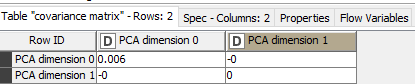# Calculate Mahalanobis distance

Hello everybody,
i have a little problem, i wanted to calculate the mahalanobis distance. but i’m not sure if it’s right.

In the beginning I reduced the four parameters to two dimensions ,

then I calculated covariance matrix ,at the end I calculated the Mohalanobis distance. but the results look like this

why there are several values under the square bracket and not only two values, i have only two dimensional values. that means the vector must have only two values.
someone can help me
thank you very much
Anass

Have you looked at the node and the workflow over on the hub?

1 Like

Hey quaeler,
yes, I have read, but I do not understand my results

You’re calculating the distance between the data in each row and the data in every other row. The end result is a matrix of distances.

2 Likes

Thank you very much elsamuel

1 Like

This topic was automatically closed 182 days after the last reply. New replies are no longer allowed.